Courses

# Doc: Angular Momentum Class 11 Notes | EduRev

## JEE : Doc: Angular Momentum Class 11 Notes | EduRev

The document Doc: Angular Momentum Class 11 Notes | EduRev is a part of the JEE Course Physics For JEE.
All you need of JEE at this link: JEE

8. Angular Momentum

8.1 Angular momentum of a particle about a point.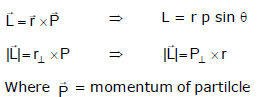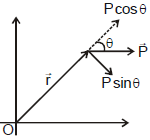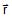= position of vector of particle with respect to point about which

angular momentum is to be calculated.

θ = angle between vectors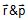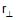= perpendicular distance of line of motion of particle from point O.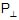= perpendicular component of momentum.

SI unit of angular momentum is kgm2/sec.

Ex.28 A particle of mass m is moving along the line y = b, z = 0 with constant speed v. State whether the angular momentum of particle about origin is increasing, decreasing or constant.

Sol.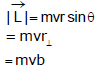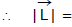constant as m, v and b all are constants.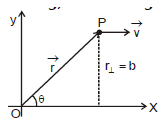Direction of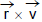also remains the same. Therefore, angular momentum of particle about origin remains constant with due course of time.

Note : In this problem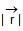is increasing, q is decreasing but r sin q, i.e., b remains constant. Hence, the angular momentum remains constant.

Ex.29 A particle of mass m is projected with velocity v at an angle q with the horizontal. Find its angular momentum about the point of projection when it is at the highest point of its trajectory.

Sol. At the highest point it has only horizontal velocity vx = v cos q. Length of the perpendicular to the horizontal velocity from 'O' is the maximum height, where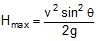⇒ Angular momentum L =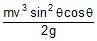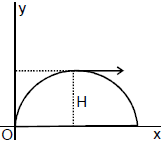8.2 Angular Momentum of a rigid body rotating about a fixed axis

Suppose a particle P of mass m is going in a circle of radius r and at some instant the speed of the particle is v. For finding the angular momentum of the particle about the axis of rotation, the origin may be chosen anywhere on the axis. We choose it at the centre of the circle. In this case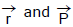are perpendicular to each other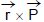and is along the axis. Thus, component of along the axis is mvr itself. The angular momentum of the whole rigid body about AB is the sum of components of all particles, i.e.,

L =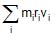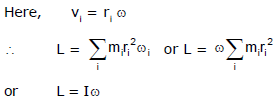Here, I is the moment of inertia of the rigid body about AB.

Note : Angular momentum about axis is the component of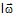along the axis. In most of the cases angular momentum about axis is Iω.

Ex.30 Two small balls A and B, each of mass m, are attached rigidly to the ends of a light rod of length d. The structure rotates about the perpendicular bisector of the rod at an angular speed w. Calculate the angular momentum of the individual balls and of the system about the axis of rotation.

Sol.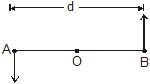Consider the situation shown in figure. The velocity of the ball A with respet to the centre O is v =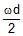.

The angular momentum of the ball with respect to the axis is

L1 = mvr =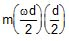=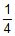mwd2. The same the angular momentum L2 of the second ball. The angular momentum of the system is equal to sum of these two angular momenta i.e., L = 1/2 mwd2.

9. Conservation of Angular momentum :

The time rate of change of angular momentum of a particle about some referenence point in an inertial frame of reference is equal to the net torques acting on it.

or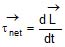....(i)

Now, suppose that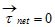, then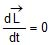, so that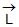= constant.

"When the resultant external torque acting on a system is zero, the total vector angular momentum of the system remains constant. This is the principle of the conservation of angular momentum.

For a rigid body rotating about an axis (the z-axis, say) that is fixed in an inertial reference frame, we have

Lz = Iw

It is possible for the moment of inertia I of a rotating body to change by rearrangement of its parts. If no net external torque acts, then Lz must remains constant and if I does change, there must be a compensating change in w. The principle of conservation of angular momentum in this case is expressed.

Iw = constant.

Ex.31 A wheel of moment of inertia I and radius R is rotating about its axis at an angular speed w0. It picks up a stationary particle of mass m at its edge. Find the new angular speed of the wheel.

Sol. Net external torque on the system is zero. Therefore, angular momentum will remain conserved. Thus,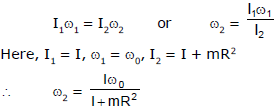Note :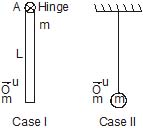Comments on Linear Momentum :

In case I : Linear momentum is not conserved just before and just after collision because during collision hinge force act as an external force.

In case II : Linear momentum is conserved just before and just after collision because no external force on the string.

Comments on Angular Momentum :

In case I : Hinge force acts at an external force during collision but except point A all the other reference point given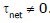. So angular momentum is conserved only for point A.

In case II : angular momentum is conserved at all points in the world.

Ex.32 A uniform rod of mass m and length l can rotate freely on a smooth horizontal plane about a vertical axis hinged at point H. A point mass having same mass m coming with an initial speed u perpendicular to the rod, strikes the rod in-elastically at its free end. Find out the angular velocity of the rod just after collision?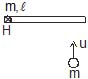Sol. Angular momentum is conserved about H because no external force is present in horizontal plane which is producing torque about H.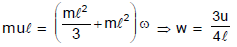Ex.33 A uniform rod of mass m and length l can rotate freely on a smooth horizontal plane about a vertical axis hinged at point H. A point mass having same mass m coming with an initial speed u perpendicular to the rod, strikes the rod and sticks to it at a distance of 3l/4 from hinge point. Find out the angular velocity of the rod just after collision?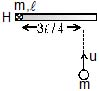Sol.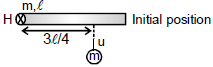from angular momentum conservation about H

initial angular momentum = final angular momentum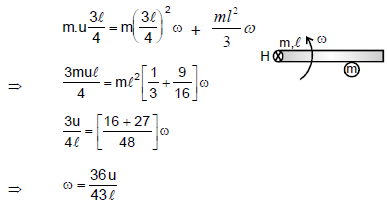Ex.34 A uniform rod AB of mass m and length 5a is free to rotate on a smooth horizontal table about a pivot through P, a point on AB such that AP = a. A particle of mass 2m moving on the table strikes AB perpendicularly at the point 2a from P with speed v, the rod being at rest. If the coefficient of restitution between them is, find their speeds immediately after impact.

Sol. Let the point of impact be Q so that

PQ = 2a

Let P be the point of pivot that AP = a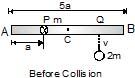Let the velocities of point, Q and the particle after impact be vq and vp respectively then from momentum conservation about point P.

Li = Lf

Offer running on EduRev: Apply code STAYHOME200 to get INR 200 off on our premium plan EduRev Infinity!

## Physics For JEE

188 videos|458 docs|276 tests

,

,

,

,

,

,

,

,

,

,

,

,

,

,

,

,

,

,

,

,

,

;